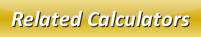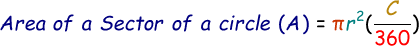# Area of a Sector of a Circle Calculator

We can calculate the area of a sector of a circle when we know the central angle and radius of the circle.

Formula to calculate area of a sector of a circle is given below:where,
A = Area of a sector of a circle
C = Central angle of a circle
r = Radius of the circle

In the below area of a sector of a circle calculator, enter the central angle and radius in the respective input boxes and click calculate button to find the answer.

 Radius of the Circle (R): Central Angle (C): [degrees] Area of a Sector of a Circle (A):

Latest Calculator Release

Average Acceleration Calculator

Average acceleration is the object's change in speed for a specific given time period. ...

Free Fall Calculator

When an object falls into the ground due to planet's own gravitational force is known a...

Torque Calculator

Torque is nothing but a rotational force. In other words, the amount of force applied t...

Average Force Calculator

Average force can be explained as the amount of force exerted by the body moving at giv...

Angular Displacement Calculator

Angular displacement is the angle at which an object moves on a circular path. It is de...